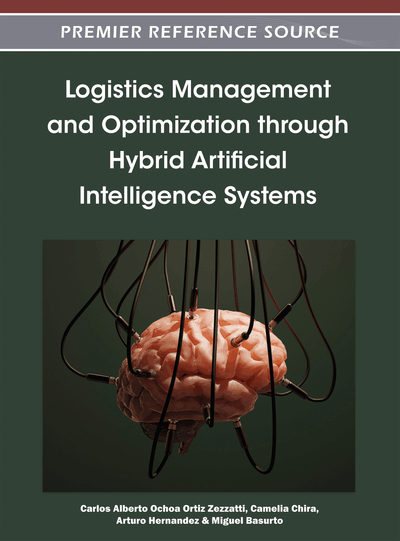# An Evolutionary Algorithm for Graph Drawing with a Multiobjective Approach

Sergio Enríquez Aranda (Autonomous University of Aguascalientes, Mexico), Eunice E. Ponce de León Sentí (Autonomous University of Aguascalientes, Mexico), Elva Díaz Díaz (Autonomous University of Aguascalientes, Mexico), Alejandro Padilla Díaz (Autonomous University of Aguascalientes, Mexico), María Dolores Torres Soto (Autonomous University of Aguascalientes, Mexico), Aurora Torres Soto (Autonomous University of Aguascalientes, Mexico) and Carlos Alberto Ochoa Ortiz Zezzatti (Juarez City University, México)
DOI: 10.4018/978-1-4666-0297-7.ch005

## Abstract

In this chapter a hybrid algorithm is constructed, implemented and tested for the optimization of graph drawing employing a multiobjective approach. The multiobjective optimization problem for graph drawing consists of three objective functions: minimizing the number of edge crossing, minimizing the graph area, and minimizing the aspect ratio. The population of feasible solutions is generated using a hybrid algorithm and at each step a Pareto front is calculated. This hybrid algorithm combines a global search algorithm (EDA — Estimation of Distribution Algorithm) with a local search Algorithm (HC — Hill Climbing) in order to maintain a balance between the exploration and exploitation. Experiments were performed employing planar and non-planar graphs. A quality index of the obtained solutions by the hybrid MOEA-HCEDA (Multiobjective Evolutionary Algorithm - Hill Climbing & Univariate Marginal Distribution Algorithm) is constructed based on the Pareto front defined in this chapter. A factorial experiment using the algorithm parameters was performed. The factors are number of generations and population size, and the result is the quality index. The best combination of factors levels is obtained.
Chapter Preview
Top

## Introduction

Graph drawing problems are a particular class of combinatorial optimization problems whose goal is to find plane layout of an input graph in such a way that certain objective functions are optimized. A large number of relevant problems in different domains can be formulated as graph layout problems. Among these problems are optimization of networks for parallel computer architectures, VLSI circuit design, information retrieval, numerical analysis, computational biology, graph theory, scheduling and archaeology. Most interesting graph drawing problems are NP-hard and their decisional versions are NP-complete (Garey & Johnson, 1983), but, for most of their applications, feasible solutions with an almost optimal cost are sufficient. As a consequence, approximation algorithms and effective heuristics are welcome in practice (Díaz et al., 2002).

Visualization of complex conceptual structures is a support tool used on several engineering and scientific applications. A graph is an abstract structure used to model information. Graphs are USD to represent information that can be modeled as connections between variables, and so, to draw graphs to put information in an understandable way.

The usefulness of graphs visualization systems depends on how easy is to catch its meaning, and how fast and clear is to interpret it. This characteristic can be expressed through of aesthetic criterions (Sugiyama, 2002; Di Battista et al., 1999) as the edges’ crossing minimization, the reduction of drawing area and the minimization of aspect ratio deployment, among others. When a graph is drawn, several aesthetic criterions have to be taken into account in order to make the graph easily readable. Planarity of a graph (graph without crosses), reduction of the drawing area, and the draw aspect ratio minimization are frequently very desirable characteristics (Di Battista et al., 1999) on graph visualization applications (Tamassia et al., 1988; Huang et al., 2000).

In order to enhance the legibility of the graph drawing is important to keep as low as possible the number of crosses as well as to keep a good aspect ratio in the draw. Another point is to maintain symmetric the drawing region (same drawing height and width). Also it is very desirable, to keep the drawing area small. This last requirement avoids the waste of screen space.

These objectives are in conflict with each other. Different objectives can produce conflict of interests (Deb, 2001). These kinds of problems are becoming very common nowadays because they are present in a wide variety of disciplines. This kind of problems has been named “multiobjective problems” and can be solved by mathematical programming (Miettinen, 1999) or by metaheuristics (Coello et al., 2009).

In order to make a graph legible (easily readable), several in conflict objectives have to be considered in the drawing, therefore the graph drawing problem can be formalized as a multiobjective problem (for example the graph drawing on a minimum area, the graph drawing with a minimum number of crossing edges and a good aspect ratio), so that, is important to establish a commitment among objectives in order to solve the different graph drawing problems. This perspective let us see that the graph drawing problem is inherently multiobjective (Enríquez, 2009).

In this chapter the following in conflict objectives have been considered:

• Minimization of the number of crossing edges in the graph: The total number of crossing edges of the graph has to be minimized.

• Minimization of the graph area: to minimize the total space used by the graph.

• Minimization of the graph aspect ratio: the graph has to be visualized in a perfect square area.

To reach the minimum crossing edges in the graph drawing is frequently to need a bigger area. At the same time, for minimizing the aspect ratio of the graph is needed to draw the nodes in a symmetrically delimited region.

## Complete Chapter List

Search this Book:
Reset